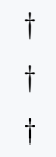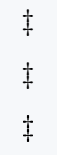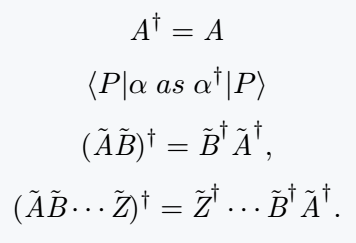# How do you make a dagger(†, and ‡) symbol in LaTeX?

Defined \dag and \dagger commands for dagger symbols and \textdagger commands for special text mode.

Symbol Dagger
Type of symbol Mathematics (Matrix)
Package No
Argument No
Latex command \dag
Example \dag → †
\documentclass{article}
\begin{document}
$\dag$\\ [6pt]
$\dagger$\$6pt] % Dagger use in text mode \textdagger \end{document} Output :## Double dagger symbol Use of double dagger symbol is much less. And different packages have the same command. But, there are morphological differences between the symbols. \documentclass{article} \begin{document}  \ddag \\ [6pt]  \ddagger \\[6pt] % Double dagger use in text mode \textdaggerdbl \end{document} Output :## Use of dagger symbol as superscript The dagger symbol is mathematically used as a hermitian adjoint. Below are various examples with the help of latex. \documentclass{article} \begin{document} \[ \textit{A}^\dag = \textit{A}$
$\langle P|\alpha\; as \; \alpha^\dag |P\rangle$
$(\tilde{\textit{A}} \tilde{\textit{B}})^\dag = \tilde{\textit{B}}^\dag \tilde{\textit{A}}^\dag ,$
$(\tilde{\textit{A}} \tilde{\textit{B}} \cdots \tilde{\textit{Z}})^\dag = \tilde{\textit{Z}}^\dag \cdots \tilde{\textit{B}}^\dag \tilde{\textit{A}}^\dag .$
\end{document}

Output :#### Md Jidan Mondal

LaTeX expert with over 10 years of experience in document preparation and typesetting. Specializes in creating professional documents, reports, and presentations using LaTeX.